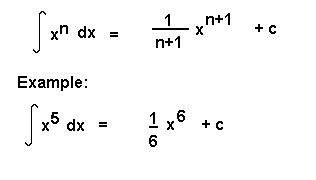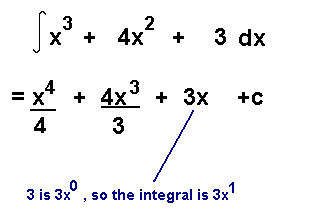## Integration

Integration is the reverse of differentiation.

However:

If y = 2x + 3, dy/dx = 2
If y = 2x + 5, dy/dx = 2
If y = 2x, dy/dx = 2

So the integral of 2 can be 2x + 3, 2x + 5, 2x, etc.
For this reason, when we integrate, we have to add a constant. So the integral of 2 is 2x + c, where c is a constant.
A "S" shaped symbol is used to mean the integral of, and dx is written at the end of the terms to be integrated, meaning "with respect to x". This is the same "dx" that appears in dy/dx .

To integrate a term, increase its power by 1 and divide by this figure. In other words:When you have to integrate a polynomial with more than 1 term, integrate each term. So: